# CAT 2019 Question Paper | Quants Slot 1

###### CAT Previous Year Paper | CAT Quants Questions | Question 8

CAT previous year papers are known to have questions that layer 2 or 3 concepts from different topics. This question fits into that bucket where it uses multiple concepts including Co-ordinate geometry, Circles & Triangles. It is a tricky problem that may end up as a time guzzler. Hence it is important to attempt these questions only if you have focussed on geometry as part of your CAT online preparation.

Question 8 : Let T be the triangle formed by the straight line 3x + 5y - 45 = 0 and the coordinate axes. Let the circumcircle of T have radius of length L, measured in the same unit as the coordinate axes. Then, the integer closest to L is [TITA]

## Best CAT Coaching in Chennai

#### CAT Coaching in Chennai - CAT 2022Limited Seats Available - Register Now!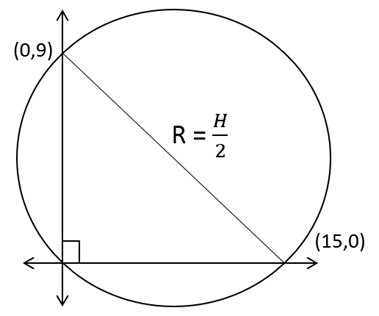We know that the equation of the straight line is 3x + 5y = 45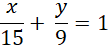The intercepts are (15,0) and (0,9) respectively
Since it's a right-angled triangle, we know that Circumradius (R) =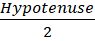Circumradius =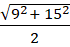=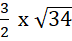We know that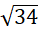is approximately equal to 6
So, from trial and error to find the closest number, we find that the value of Circumradius is very close to 9
So, the integer closest to L = 9

The question is "Let T be the triangle formed by the straight line 3x + 5y - 45 = 0 and the coordinate axes. Let the circumcircle of T have radius of length L, measured in the same unit as the coordinate axes. Then, the integer closest to L is [TITA] "

##### Hence, the answer is 9

###### CAT Coaching in ChennaiCAT 2023

Classroom Batches Starting Now! @Gopalapuram

###### Best CAT Coaching in Chennai Introductory offer of 5000/-

Attend a Demo Class

##### Where is 2IIM located?

2IIM Online CAT Coaching
A Fermat Education Initiative,
58/16, Indira Gandhi Street,
Kaveri Rangan Nagar, Saligramam, Chennai 600 093

##### How to reach 2IIM?

Mobile: (91) 99626 48484 / 94459 38484
WhatsApp: WhatsApp Now
Email: info@2iim.com